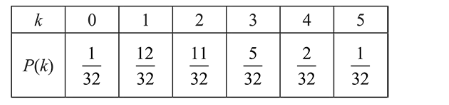# Ap unbiased coin is tossed 5 times.Question:

Ap unbiased coin is tossed 5 times. Suppose that a variable

$X$ is assigned the value $k$ when $k$ consecutive heads are obtained for $k=3,4,5$, otherwise $X$ takes the value $-1$.

Then the expected value of $X$, is:

1. (1) $\frac{3}{16}$

2. (2) $\frac{1}{8}$

3. (3) $-\frac{3}{16}$

4. (4) $-\frac{1}{8}$

Correct Option: , 2

Solution:$k=$ No. of times head occur consecutively

Now expectation

$=\sum x P(k)=(-1) \times \frac{1}{32}+(-1) \times \frac{12}{32}+(-1) \times \frac{11}{32}$

$+3 \times \frac{5}{32}+4 \times \frac{2}{32}+5 \times \frac{1}{32}=\frac{1}{8}$